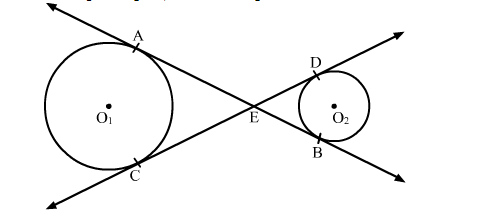# In the given figure, common tangents AB and CD to the two circle with centres`
Question:

In the given figure, common tangents AB and CD to the two circle with centres O1 and O2 intersect at E. Prove that AB = CDSolution:

We know that tangent segments to a circle from the same external point are congruent.
So, we have
EA = EC for the circle having centre O1
and
ED = EB for the circle having centre O1
Now, Adding ED on both sides in EA = EC, we get
EA + ED = EC + ED
⇒EA + EB = EC + ED
⇒AB = CD